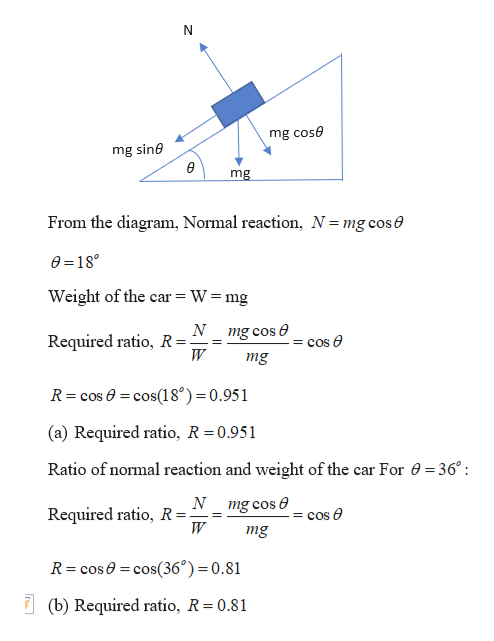# A car is traveling up a hill that is inclined at an angle θ above the horizontal. Determine the ratio of the magnitude of the normal force to the weight of the car when (a)  θ = 18o and (b)  θ = 36o.

Question
15 views

A car is traveling up a hill that is inclined at an angle θ above the horizontal. Determine the ratio of the magnitude of the normal force to the weight of the car when (a)  θ = 18o and (b)  θ = 36o.

check_circle

Step 1

Given:

Angle of inclination for part a = 18 degrees

Angle of inclination for part b = 36 degrees

Step 2

Calculating the ratio of normal reaction and weight ...help_outlineImage Transcriptionclosemg cose mg sine mg From the diagram, Normal reaction, N = mg cose e 18 Weight of the car = W = mg N mg cos cos e Required ratio, R=. mg R cos cos18°)=0.951 (a) Required ratio, R = 0.951 Ratio of normal reaction and weight of the car For 0 = 36° mg cos Required ratio, R= W cos e = mg R cose cos(36°)=0.81 (b) Required ratio, R = 0.81 fullscreen

### Want to see the full answer?

See Solution

#### Want to see this answer and more?

Solutions are written by subject experts who are available 24/7. Questions are typically answered within 1 hour.*

See Solution
*Response times may vary by subject and question.
Tagged in

### Newtons Laws of Motion